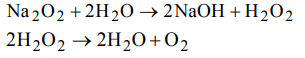## Hydrogen Questions and Answers Part-1

1. $H_{2}O_{2}$  is commonly prepared in lab. by the reaction of
a) $PbO_{2}+H_{2}SO_{4}$
b) $MnO_{2}+H_{2}SO_{4}$
c) $BaO_{2}+H_{2}O+CO_{2}$
d) $Na_{2}O_{2}+H_{2}O$

Explanation: $BaO_{2}+H_{2}O+CO_{2}$    $\rightarrow$ $BaCO_{3}+H_{2}O_{2}$   (Merck process)

2.Calculate the normality of 10 volume $H_{2}O_{2}$
a) 1.7 N
b) 12 N
c) 30.3 N
d) 0.0303 N

Explanation:3. Commercial 10 volume $H_{2}O_{2}$  is a solution with a strength of approximately
a) 30%
b) 3%
c) 1%
d) 10%

Explanation:4. A 6 volume sample of $H_{2}O_{2}$
a) will contain 6% V/V of $H_{2}O_{2}$
b) will contain 6% W/V of $H_{2}O_{2}$
c) would give 6 volumes of oxygen per unit volume of $H_{2}O_{2}$  sample at STP
d) would give 6 volumes of oxygen per unit weight of $H_{2}O_{2}$  sample at STP

Explanation: 6 volume H2O2 would give 6 volumes of O2 per unit volume of H2O2

5. The structure of $H_{2}O_{2}$  is
a) planar
b) non planar
c) spherical
d) linear

Explanation: Structure of H2O2 is nonplanar

6. In which of the following reactions, $H_{2}O_{2}$ is acting as a reducing agent
a) $H_{2}O_{2}+SO_{2}\rightarrow H_{2}SO_{4}$
b) $2KI+H_{2}O_{2}\rightarrow2KOH+ I_{2}$
c) $PbS+4H_{2}O_{2}\rightarrow PbSO_{4}+4 H_{2}O$
d) $Ag_{2}O+H_{2}O_{2}\rightarrow 2Ag+ H_{2}O+O_{2}$

Explanation: SO2 changes to H2 SO4 (O.N. changes from +4 to +6 oxidation)7. Which of the following is formed by the action of water on sodium peroxide
a) $H_{2}$
b) $N_{2}$
c) $O_{2}$
d) $CO_{2}$

Explanation:8. The percentage by weight of hydrogen in $H_{2}O_{2}$   is
a) 5.88
b) 6.25
c) 25
d) 50

Explanation:9. In which of the following reactions, $H_{2}O_{2}$  acts as a reducing agent?
a) $PbO_{2}\left(s\right)+H_{2}O_{2}\left(aq\right)\rightarrow PbO\left(s\right)+ H_{2}O\left(l\right)+O_{2}\left(g\right)$
b) $Na_{2}SO_{3}\left(aq\right)+H_{2}O_{2}\left(aq\right)\rightarrow Na_{2}SO_{4}\left(aq\right)+ H_{2}O\left(l\right)$
c) $2KI\left(aq\right)+H_{2}O_{2}\left(aq\right)\rightarrow 2KOH\left(aq\right)+ I_{2}\left(s\right)$
d) $KNO_{2}\left(aq\right)+H_{2}O_{2}\left(aq\right)\rightarrow KNO_{3}\left(aq\right)+ H_{2}O\left(l\right)$

Explanation: PbO2 $\rightarrow$ PbO (change in O.S. is +4 to +2 hence reduction)